## Course content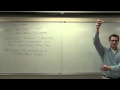0h17m

### Statistics Lecture 1.1: The Key Words and Definitions For Elementary Statistics

Statistics Lecture 1.1: The Key Words and Definitions For Elementary Statistics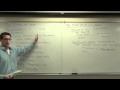0h31m

### Statistics Lecture 1.3: Exploring Categories of Data, Levels of Measurement

Statistics Lecture 1.3: Exploring Categories of Data, Levels of Measurement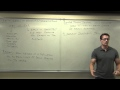0h31m

### Statistics Lecture 1.5: Sampling Techniques. How to Develop a Random Sample

Statistics Lecture 1.5: Sampling Techniques. How to Develop a Random Sample1h07m

### Statistics Lecture 2.2: Creating Frequency Distribution and Histograms

Statistics Lecture 2.2: Creating Frequency Distribution and Histograms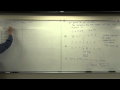1h11m

### Statistics Lecture 3.2: Finding the Center of a Data Set. Mean, Median, Mode

Statistics Lecture 3.2: Finding the Center of a Data Set. Mean, Median, Mode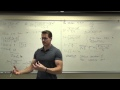1h56m

### Statistics Lecture 3.3: Finding the Standard Deviation of a Data Set

Statistics Lecture 3.3: Finding the Standard Deviation of a Data Set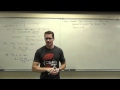1h31m

### Statistics Lecture 3.4: Finding Z-Score, Percentiles and Quartiles, and Comparing Standard Deviation

Statistics Lecture 3.4: Finding the Z-Score, Percentiles and Quartiles, and Comparing Standard Deviation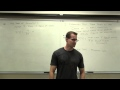1h42m

### Statistics Lecture 4.2: Introduction to Probability

Statistics Lecture 4.2: Introduction to Probability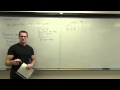1h09m

### Statistics Lecture 4.3: The Addition Rule for Probability

Statistics Lecture 4.3: The Addition Rule for Probability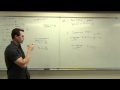1h06m

### Statistics Lecture 4.4: The Multiplication Rule for "And" Probabilities.

Statistics Lecture 4.4: The Multiplication Rule for "And" Probabilities.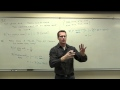0h32m

### Statistics Lecture 4.5: Probability of Complementary Events with "At Least One"

Statistics Lecture 4.5: Probability of Complementary Events with "At Least One"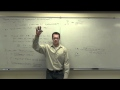1h37m

### Statistics Lecture 4.7: Fundamental Counting Rule, Permutations and Combinations

Statistics Lecture 4.7: Fundamental Counting Rule, Permutations and Combinations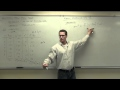1h12m

### Statistics Lecture 5.2: A Study of Probability Distributions, Mean, and Standard Deviation

Statistics Lecture 5.2: A Study of Probability Distributions, Mean, and Standard Deviation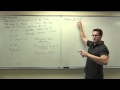1h32m

### Statistics Lecture 5.3: A Study of Binomial Probability Distributions

Statistics Lecture 5.3: A Study of Binomial Probability Distributions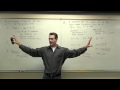0h42m

### Statistics Lecture 5.4: Finding Mean and Standard Deviation of a Binomial Probability Distribution

Statistics Lecture 5.4: Finding the Mean and Standard Deviation of a Binomial Probability Distribution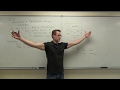2h11m

### Statistics Lecture 6.2: Introduction to the Normal Distribution and Continuous Random Variables

Statistics Lecture 6.2: Introduction to the Normal Distribution and Continuous Random Variables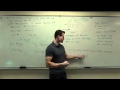0h56m

### Statistics Lecture 6.3: The Standard Normal Distribution. Using z-score, Standard Score

Statistics Lecture 6.3: Applications of the Standard Normal Distribution. Using z-score, Standard Score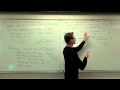0h50m

### Statistics Lecture 6.4: Sampling Distributions Statistics. Using Samples to Approx. Populations

Statistics Lecture 6.4: Sampling Distributions of Sample Statistics. Using Samples to Approx. Populations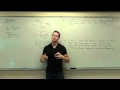1h31m

### Statistics Lecture 6.5: The Central Limit Theorem for Statistics. Using z-score, Standard Score

Statistics Lecture 6.5: The Central Limit Theorem for Statistics. Using z-score, Standard Score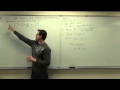2h24m

### Statistics Lecture 7.2: Finding Confidence Intervals for the Population Proportion

Statistics Lecture 7.2: Finding Confidence Intervals for the Population Proportion